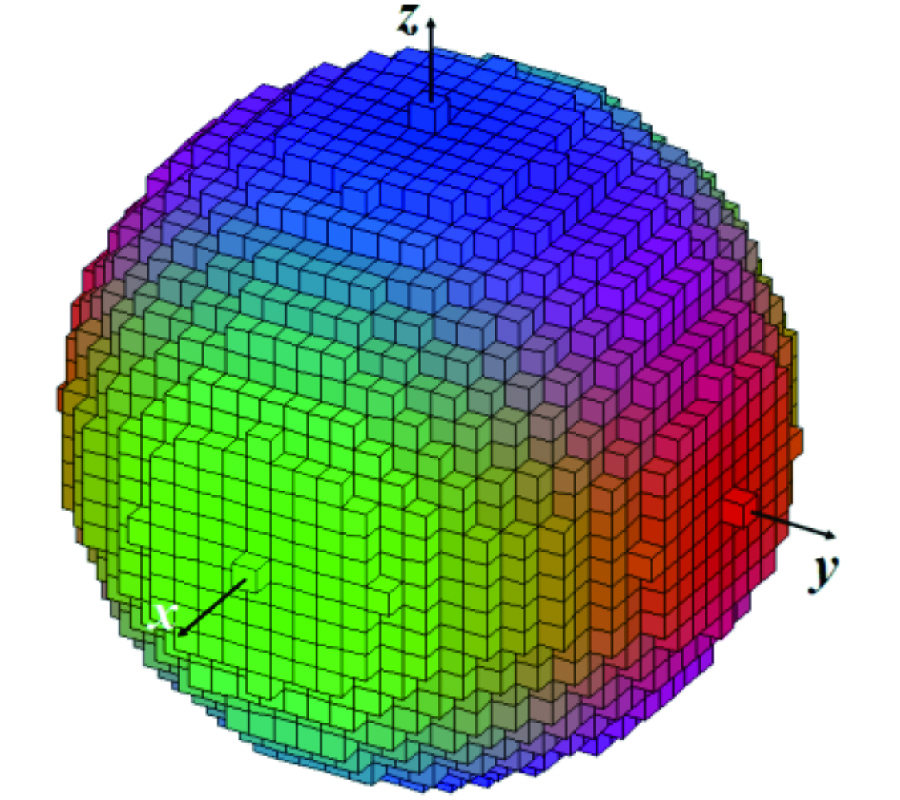Vol. 67
Latest Volume
All Volumes
All Journals
2007-01-11
PIER
Vol. 67, 341-355, 2007
An Efficient Algorithm for EM Scattering by Electrically Large Dielectric Objects Using Mr-Qeb Iterative Scheme and Cg-FFT Method
Lei Zhao , Tie-Jun Cui and Wei-Dong Li
In this paper, an efficient algorithm is presented to analyze the electromagnetic scattering by electrically large-scale dielectric objects. The algorithm is based on the multi-region and quasiedge buffer (MR-QEB) iterative scheme and the conjugate gradient (CG) method combined with the fast Fourier transform (FFT). This algorithm is done by dividing the computational domain into small sub-regions and then solving the problem in each sub-region with buffer area using the CG-FFT method. Considering the spurious edge effects, local quasi-edge buffer regions are used to suppress these unwanted effects and ensure the stability. With the aid of the CG-FFT method, the proposed algorithm is very efficient, and can solve very largescale problems which cannot be solved using the conventional CG-FFT method in a personal computer. The accuracy and efficiency of the proposed algorithm are verified by comparing numerical results with analytical Mie-series solutions for dielectric spheres.2006-11-10
PIER
Vol. 67, 329-339, 2007
Microstrip Nonuniform Impedance Resonators
In this paper, a new structure is introduced to design resonators with very short length and without any discontinuity.The introduced structure, called Nonuniform Impedance Resonator (NIR), is an open- or short-circuited Nonuniform Transmission Line (NTL).To synthesize NIRs, strip width or the characteristic impedance function of the microstrip NIRs is expanded in a truncated Fourier series, first. Then, the optimum values of the coefficients of the series are obtained through an optimization approach.The usefulness of the proposed method is verified using some examples.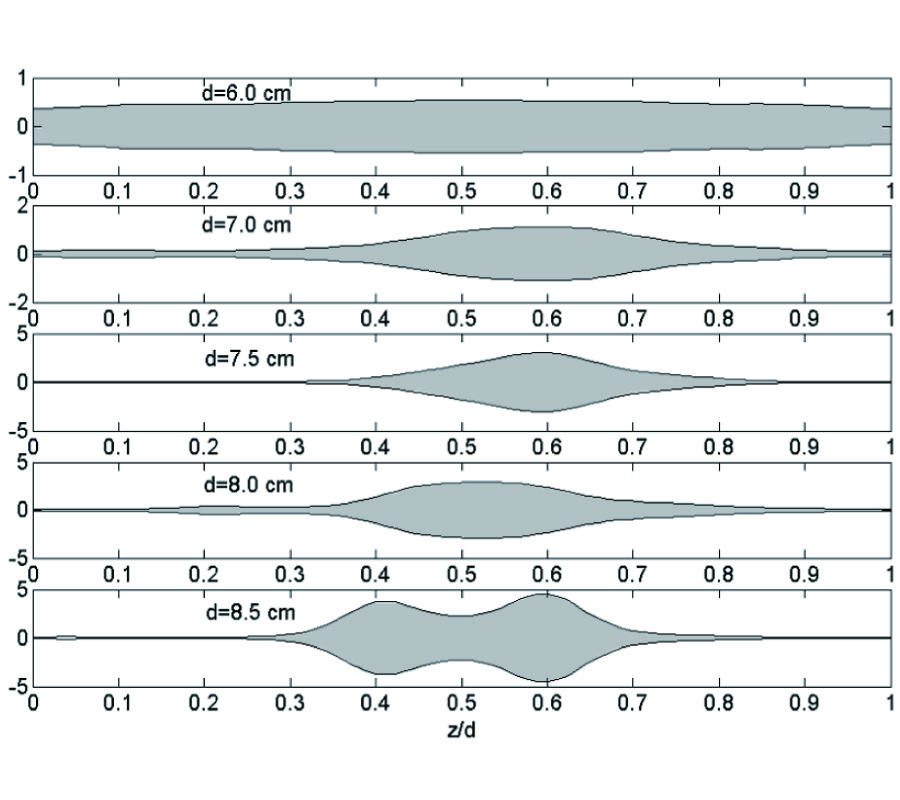2006-11-10
PIER
Vol. 67, 317-328, 2007
Backscattering from Partially Convex Targets in Free Space with h -Beam Wave Incidence
Hosam El-Ocla
In , scattering problems of plane and beam wave incidences were presented. In that study, it was found that the target configuration together with beam width have a major effect on the laser RCS (LRCS). This conclusion was proved for horizontal incident wave polarization (E-polarization) in free space. The polarization of incident waves is one of the key parameters in the scattering problems and in particular in the resonance region where the target has a comparable size with the wave length. In this work, we extend our study and investigate the impact of vertical incident wave polarization (H-polarization) on the LRCS of large size targets and compare it with RCS of targets with plane wave incidence.2006-11-10
PIER
Vol. 67, 297-316, 2007
Characteristics of an Offset Longitudinal / Transverse Slot Coupled Crossed Waveguide Junction Using Multiple Cavity Modeling Technique Considering the Te00 Mode at the Slot Aperture
Sushrut Das , Ajay Chakraborty and Ashmi Chakraborty Das
A moment method analysis of a broad wall slot coupled crossed rectangular waveguide junction has been presented in this paper. The coupling slot is longitudinal/transverse and offset from the centre lines of the guides. The integral equations, governing the characteristics of the device, have been obtained using Multiple Cavity Modeling Technique, taking into account of the finite wall thickness and the TE00 mode at the slot apertures and have been solved using Moment Method to obtain the aperture distribution. The normalized resonant lengths/complex S-parameters have been calculated from this field distribution for different guide height/slot offsets/slot widths/slot thickness of the longitudinal/transverse slot over the frequency band. Numerical data, thus obtained, have been compared with measured/literature available/CST Microwave Studio Simulated data. The theory has been validated by the reasonable agreement obtained between them. It has been shown that, neglecting the TE00 mode at the slot apertures can adversely effect the estimation of the resonant frequency and equivalent network parameters.2006-11-10
PIER
Vol. 67, 275-296, 2007
On the Spectral Efficiency of a Sphere
Mats Gustafsson and Sven Nordebo
In many cases it is desired to have both high capacity and small antennas in wireless communication systems. Unfortunately, the antenna performance deteriorates when the antennas get electrically small. In this paper, fundamental limitations from antenna theory and broadband matchingare used to analyze fundamental limitations on the spectral efficiency of an arbitrary antenna inserted inside a sphere.2006-11-10
PIER
Vol. 67, 263-274, 2007
Geometrical Analysis of High Altitude Platforms Cellular Footprint
Moawad Ibrahim Dessouky , Hamdy Sharshar and Yasser Albagory
Cellular communications using high altitude platforms will predominate the existing conventional terrestrial or satellite systems. Radio coverage of HAPs is an important issue that affect the cellular system design, therefore a deep analysis of the HAP cell footprint will be presented in two coverage models and the cell parameters are also deduced for subsequent cellular design. In the design stage, a cell generating algorithm is introduced to define their locations more accurate. When deploying the cellular system with such algorithm, the cells have proper overlap and interspacing.2006-11-10
PIER
Vol. 67, 251-261, 2007
Design of High Altitude Platforms Cellular Communications
Moawad Ibrahim Dessouky , Hamdy Sharshar and Yasser Albagory
Cellular communications using high altitude platforms (HAPs) will predominate the existing conventional terrestrial or satellite systems but requires some optimization especially in the cellular radio coverage design. The design of HAPs cellular system suffers from increasing cell area when directing the same beam to cover lower elevation regions and therefore requires some modification in its location. In this paper either spot-beam antennas or antenna phased arrays are used in the radio coverage which are optimized in directing their beams to satisfy mostly uniform cellular layout with minimal coverage problems such as coverage gaps between cells or excessive cells overlap. The cells locations determination is done through proposed algorithm that takes into consideration the cell area increase at lower elevation angles and provides mostly uniform radio coverage especially at the cell edges.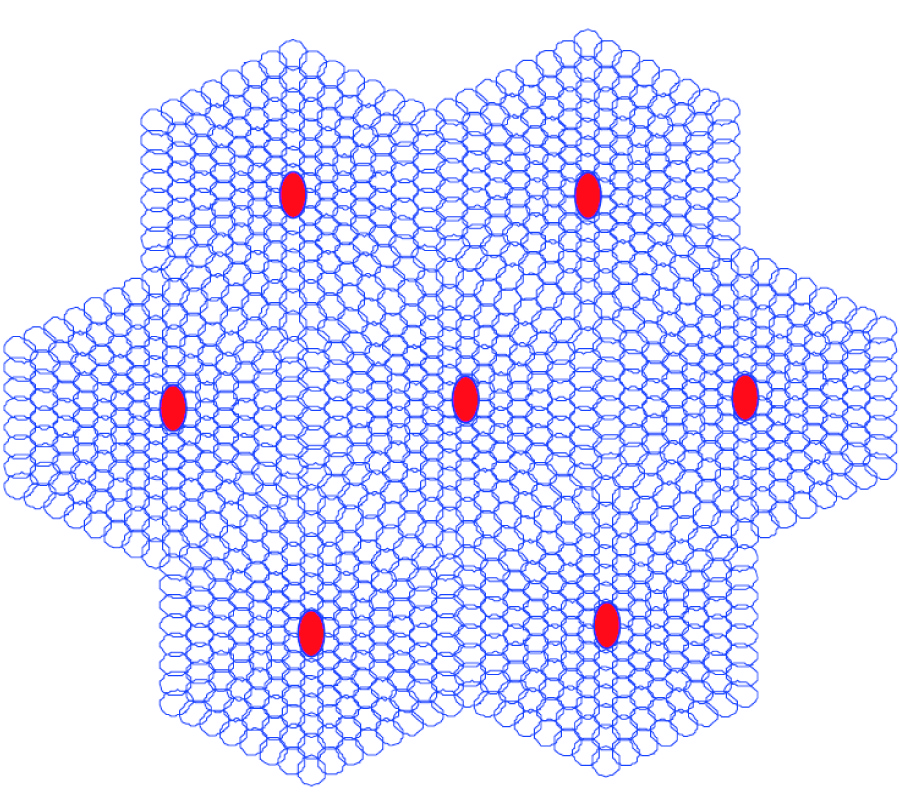2006-11-10
PIER
Vol. 67, 231-249, 2007
Augmentation of Anti-Jam GPS System Using Smart Antenna with a Simple DOA Estimation Algorithm
Mainak Mukhopadhyay , Binay Sarkar and Ajay Chakraborty
Smart Antenna system analysis presented with multipath and null constraint for reducing interference and efficient use of spectrum with the help of LMS algorithm for GPS (Global Positioning System) System. A new simple DOA (Direction of Arrival) estimation method by rotation of antenna plane proposed. Simulated Result obtained using MATLABTM.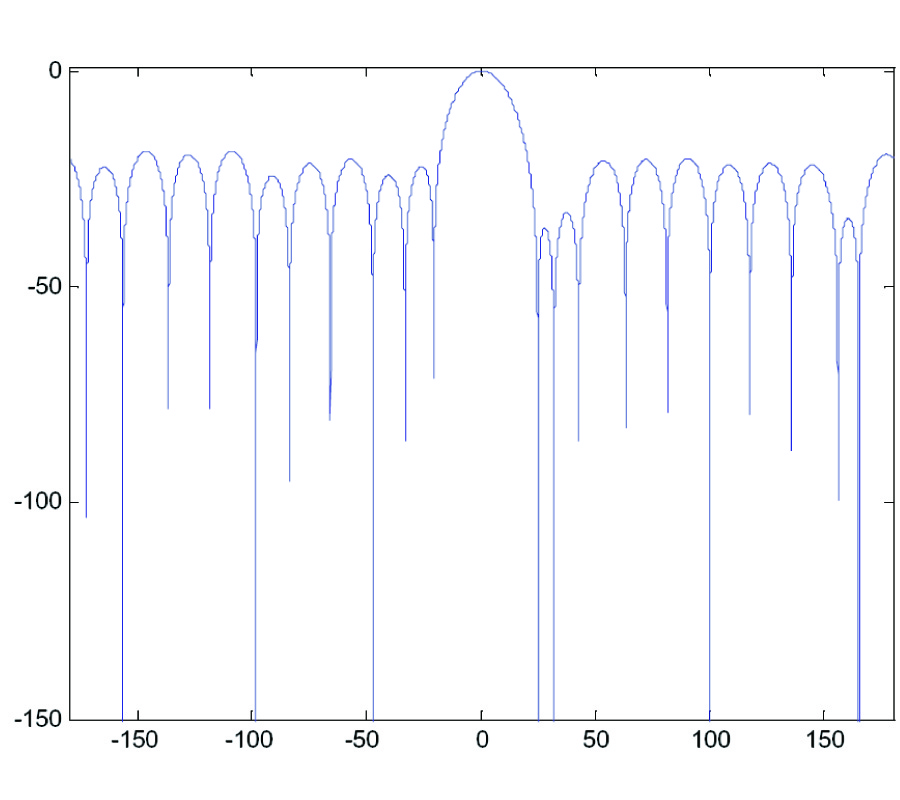2006-10-26
PIER
Vol. 67, 205-230, 2007
Application of Mode Matching Method to Analysis of Axisymmetric Coaxial Discontinuity Structures Used in Permeability and/OR Permittivity Measurement
Ruifeng Huang and Daming Zhang
This paper presents a mode matching method to analyze axisymmetric coaxial discontinuity structures, commonly used in the permeability and/or permittivity measurement.By performing the mode matching procedures at all discontinuity interfaces, a set of general simultaneous equations are derived, which can be easily solved.The s parameters and field distribution in the structures are readily obtained from the solution to the simultaneous equations. As a preliminary preparation for the mode matching method, the propagation constants of all the sections in the structure have to be solved.A one-dimensional frequency domain finite difference method is presented in this paper to efficiently solve the propagation constants for the multi-layered axisymmetric structures. Numerical examples show that the results obtained from the method in this paper are in good agreement with those from other methods in the published literature papers, and the method presented here has much higher efficiency.2006-10-26
PIER
Vol. 67, 181-203, 2007
Microwave Emission from Two-Dimensional Inhomogeneous Dielectric Rough Surfaces Based on Physics-Based Two-Grid Method
Kunshan Chen , Leung Tsang and Jian-Cheng Shi
Numerical simulations of emission for two-dimensional randomly rough surfaces with an inhomogeneous layered medium are presented. The inhomogeneous layered medium is modeled by a generalized n-layered stratified media. The numerical method was adopted from the physics-based two-grid method (PBTG). To ensure the strict accuracy requirement while to relief the memory and CPU resources, the PBTG in conjunction with the sparse-matrix canonical grid method (SMCG) was used in this paper. In so doing the reflected terms of the dyadic Green's function that accounts for layered media were added into the integral equations governing the surface tangential fields. Since the reflected part of the dyadic Green's function does not contain any singularity, the normal components of the fields remain the same as in the case of homogeneous surfaces. It was found that the elements of Green's tensor are only important to the near-field since they decay very fast as spatial distance goes beyond a few wavelengths. The resulting integral equations are then solved by the Method of Moment (MoM). Comparisons between the inhomogeneous and the homogeneous rough surfaces suggest that the presence of the inhomogeneous layered medium has non-negligible contributions to emission, depending on the dielectric gradient and is polarization dependent.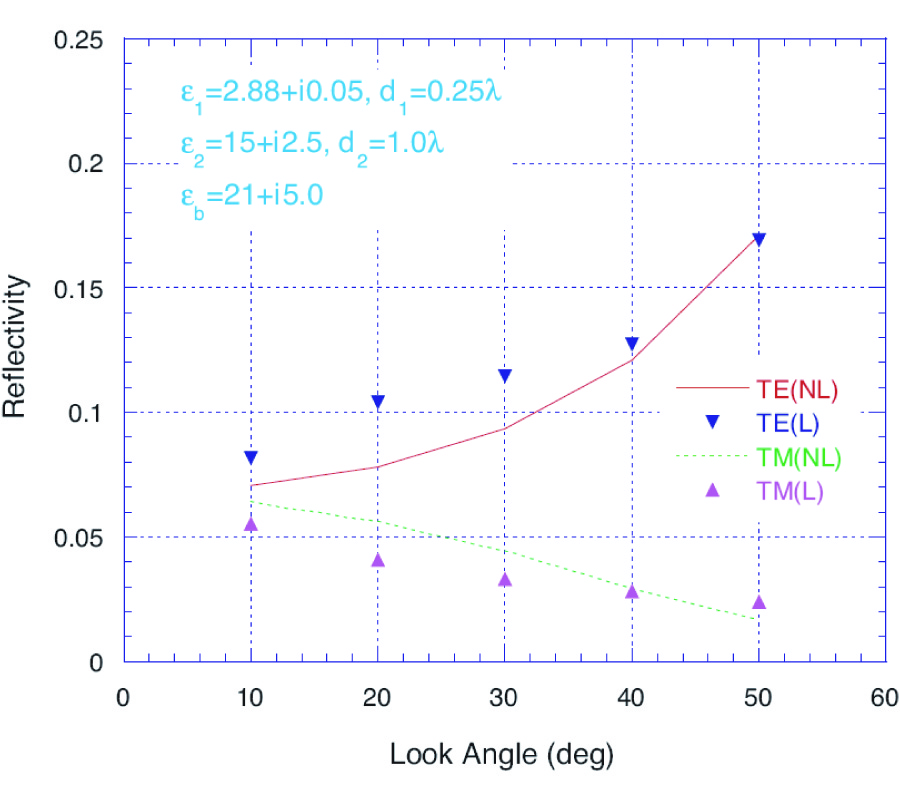2006-10-26
PIER
Vol. 67, 173-179, 2007
A Compact Microstrip Square-Ring Slot Antenna for UWB Applications
A microstrip square-ring slot antenna (MSRSA) for UWB (Ultra Wideband) antenna applications is proposed and improved by compaction. This structure is fed by a single microstrip line with a fork like-tuning stub. By splitting the square-ring slot antenna (SRSA) and optimization ofthe feeding network, the required impedance bandwidth is achieved over the UWB frequency range (3.1 to 10.6 GHz). The experimental and simulation results exhibit good agreement together. Parametric study is applied to compaction of structure. This compaction provides a good radiation pattern and a relatively constant gain over the entire band off requency.2006-10-26
PIER
Vol. 67, 153-172, 2007
Ray Tracing Radio Waves in Wildfire Environments
Kgakgamatso Mphale and Mal Heron
Wildfires are uncontrolled exothermic oxidation of vegetation. Flame combustion temperatures could be in excess of 1600 K. Under the high temperature environment, plants' organic structure crumbles to release omnipresent alkali nutrients into the combustion zone. The alkali based compounds thermally decomposed to constituent atoms which ultimately ionised to give ions and electrons. The presence of electrons in the flame lowers its refractive index, thereby creating a medium of spatially varying refractive index. In the medium, incident radio waves change speed and are consequently deflected from their original path. The refraction has an effect of decreasing signal intensity at a targeted receiver which is at the same height as a collimated beam transmitter which is at a considerable distance away from the former. A numerical experiment was set to investigate the sub refractive behaviour of a very high intensity eucalyptus wildfire (90 MWmâˆ’1) plume using two dimensional (2D) ray tracing scheme. The scheme traces radio rays as they traverse the plume. The ratio of number rays in a collimated beam reaching the targeted receiver to number of rays leaving the transmitter is used to calculate signal intensity loss in decibels (dB) at the receiver. Assuming an average natural plant alkali (potassium) content of 0.5%, attenuation (dB) was observed to be factor of both propagation frequency and temperature at the seat of the fire plume; and only of temperature at cooler parts of the plume. The 2D ray tracing scheme predicted a maximum attenuations of 14.84 and 5.47 dB for 3000 and 150 MHz respectively at 0.8 m above canopy-flame interface over propagation path of 48.25 m. An attenuation of 0.85 dB was predicted for frequencies from 150-3000 MHz over the same propagation distance at plume height of 52.8 m above ground.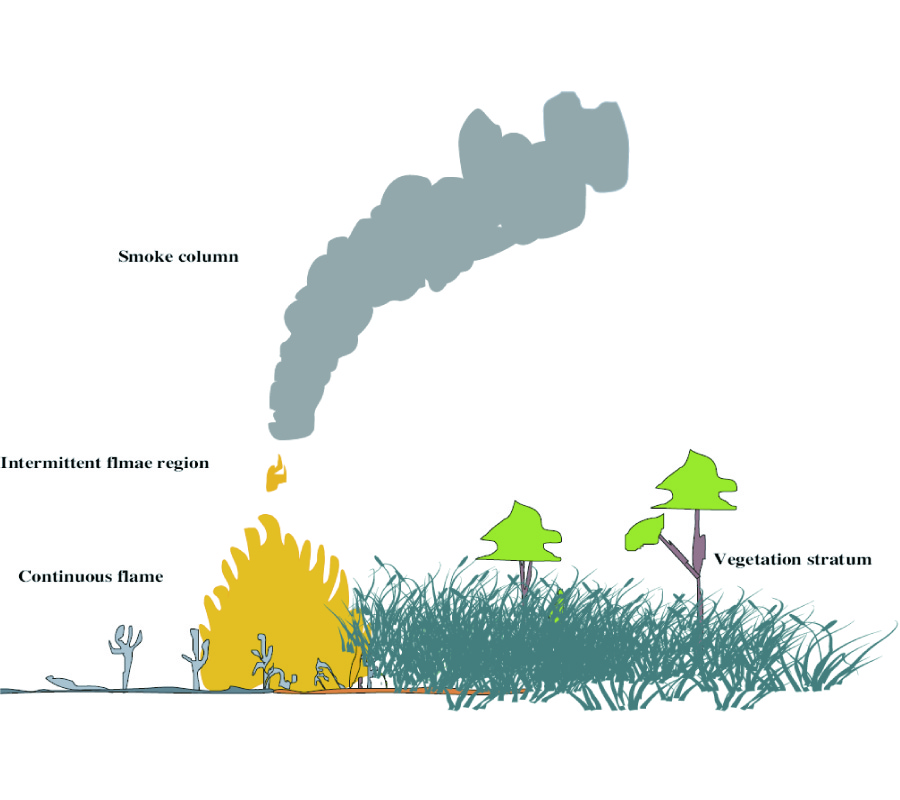2006-10-26
PIER
Vol. 67, 135-152, 2007
Analysis of Radiation from a Cylindrical-Rectanguar Microstrip Patch Antenna Loaded with a Supersrate and an Air Gap, Using the Electric Surface Current Model
Francis Cooray and John Kot
Radiation from a superstrate loaded cylindrical-rectangular microstrip patch antenna with an air gap between the substrate and the superstrate, is analyzed using the full-wave approach and the electric surface current model. Results are presented in the form of normalized radiation patterns for various thicknesses of the air gap and also for superstrates made of lossy dielectric material, to show the effects of these on the radiation from the antenna. Both axialand azimuthal current elements are considered.2006-10-26
PIER
Vol. 67, 113-134, 2007
Electromagnetic Scattering by a Metallic Spheroid Using Shape Perturbation Method
Aristides Kotsis and John Roumeliotis
The scattering of a plane electromagnetic wave by a perfectly conducting prolate or oblate spheroid is considered analytically by a shape perturbation method. The electromagnetic field is expressed in terms of spherical eigenvectors only, while the equation of the spheroidal boundary is given in spherical coordinates. There is no need for using any spheroidal eigenvectors in our solution. Analytical expressions are obtained for the scattered field and the scattering cross-sections, when the solution is specialized to small values of the eccentricity h = d/(2a), (h<<1), where d is the interfocal distance of the spheroid and 2a the length of its rotation axis. In this case exact, closed-form expressions, valid for each small h, are obtained for the expansion coefficients g(2) and g(4) in the relation S(h) = S(0)[1 + g(2)h2 + g(4)h4 + O(h6)] expressing the scattering cross-sections. Numerical results are given for various values of the parameters.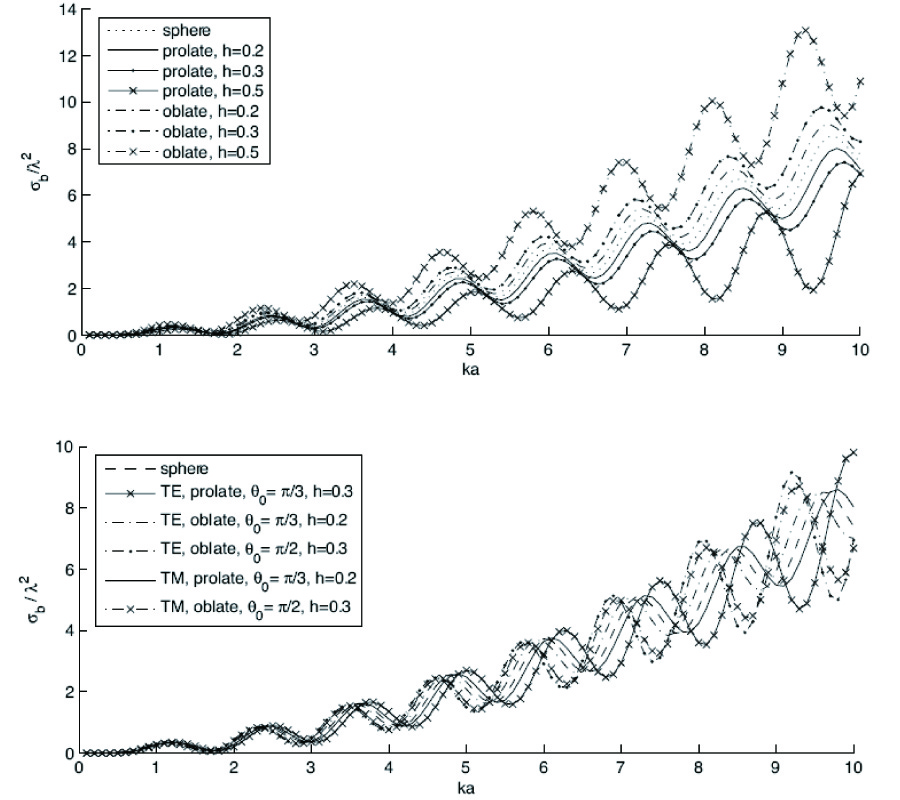2006-10-26
PIER
Vol. 67, 39-112, 2007
A Rapidly-Convergent, Mixed-Partial Derivative Boundary Condition Green's Function for an Anisotropic Half-Space: Perfect Conductor Case
John Jarem
The problem of determining the Green's function of an electric line source located in a permeable, anisotropic (Ëœμxx, Ëœμxy, Ëœμyx, Ëœμyy and Ëœεzz nonzero interacting parameters) half-space above a Perfect Magnetic Conductor (PMC) ground plane (called the TMz case herein) for the case where image theory cannot be applied to find the Green's function of the PMC ground plane system has been studied. Monzon [2, 3] studied the Green's function TEz problem dual to the present one for two cases; (1) when the system was unbounded, anisotropic space where Ëœεxx, Ëœεxy, Ëœεyx, Ëœεyy and Ëœμzz were the nonzero interacting parameters; and (2) when the scattering system was an anisotropic half-space located above a Perfect Electric Conductor (PEC) ground plane and where Ëœεxx, Ëœεyy and Ëœμzz were the nonzero interacting parameters and Ëœεxy = Ëœεyx = 0. Monzon  referred to the latter ground plane case as the case where "usual image" theory could be used to find the Green's function of the system. The Green's function for the TMz-PMC case studied herein was derived by introducing and using a novel, linear coordinate transformation, namely Ëœx = (ÏƒP /Ï„)Ëœx + ÏƒMËœy, Ëœy = Ëœy, (Eqs. (6c,d), herein). This transformation, a modification of that used by [2, 3], reduced Maxwell's anisotropic equations of the system to a non-homogeneous, Helmholtz wave equation from which the Green's function, G, meeting boundary conditions, could be determined. The coordinate transformation introduced was useful for the present PMC ground plane problem because it left the position of the PMC ground plane and all lines parallel to it, unchanged in position from the original coordinate system, thus facilitating imposition of EM boundary conditions at the PMC ground plane. In transformed (or primed) coordinates, for the TMz-PMC and TEz-PEC ground plane problems, respectively, the boundary conditions for the Green's functions GTM â‰¡ Ez and GTE â‰¡ Hz were shown to be [Î±*(âˆ‚G/âˆ‚Ëœx) + (âˆ‚G/âˆ‚Ëœy) |Ëœy=0 = 0, where G = GTM or GTE, Î± = Î±TM or Î±TE, where Î±TM and Î±TE are complex constants (dually related to each other by Ëœμ â†” Ëœε), and where Ëœy' = 0 is the position of the ground plane. An interesting result of the analysis was that the constant Î±TE (as far as the author knows) coincidently turned out to be the same as the first of two important constants, namely S1(dlv/dl) and S2(dlv/dl), which were used by Monzon [2, 3] to formulate integral equations (based on Green's second theorem) from which EM scattering from anisotropic objects could be studied. Spatial Fourier transform (k-space) techniques were used to determine the Green's function of the Helmholtz wave equation expressed in transformed coordinates which satisfied the mixed-partial derivative boundary condition of the system. The Green's function G was expressed as a sum of a "free space" Green's function gf (proportional to a Hankel function H(2)0 and assumed excited by the line source in unbounded space) and a homogeneous Green's function g whose spectral amplitude was chosen such that, when g was added to gf , the sum G = gf + g, satisfied boundary conditions. The k-space, Sommerfeld integrals making up g turned out to converge slowly, and so, using contour integration in the complex plane (Appendix B), g was expressed in a rapidly convergent form, and thus one leading to its practical numerical evaluation. Extensive numerical testing of how well the Green's functions G = gf + g satisfied boundary conditions and how well the homogeneous Green's function g satisfied Green's second theorem was performed. Excellent verification of the numerical and analytical procedures were found and displayed in the error tables of Tables 1-4 of the paper. Plots illustrating the novel coordinate transformation introduced were presented and several numerical plots of the Green's function developed herein were presented. The application of the present work to find the Green's function for the case when a negative-index, anisotropic metamaterial is adjacent to a conducting ground plane is discussed. Application of the Green's function theory developed herein to multi-layer anisotropic systems is discussed.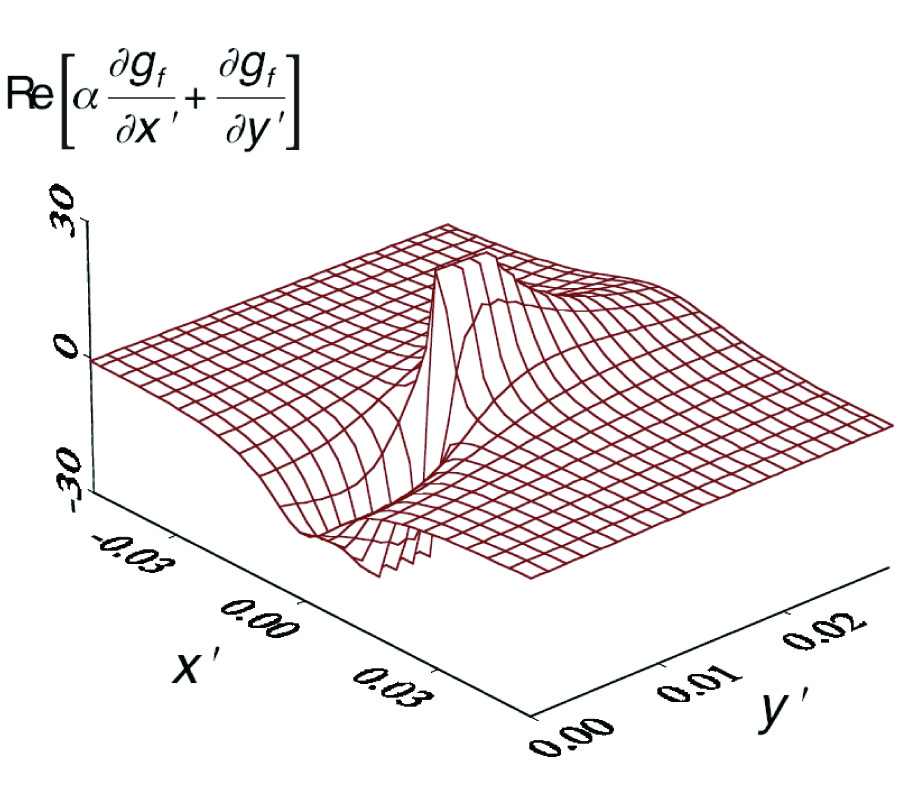2006-10-26
PIER
Vol. 67, 25-37, 2007